# Trigonometric Ratios of Multiple Angles## Trigonometric Ratios of Multiple Angles

If A is an angle, then 2A, 3A, 4A, 5A, etc are called multiple angles of A. In this section we will discuss about the trigonometric ratios of multiple angles 2A and 3A in terms of A.

********************

10 Math Problems officially announces the release of Quick Math Solver, an Android App on the Google Play Store for students around the world.

********************

### Trigonometric Ratios of Angle 2A in terms of A

#### Formula for sin2A#### Formula for cos2A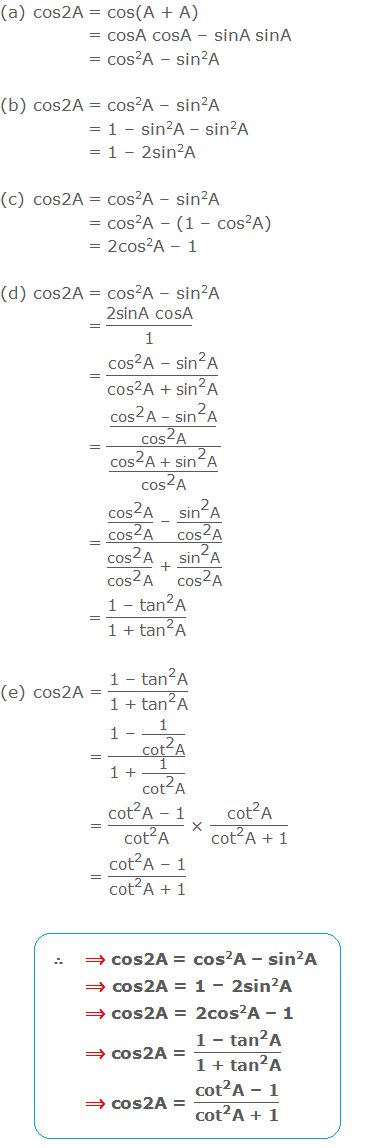#### The formula for tan2A and cot2A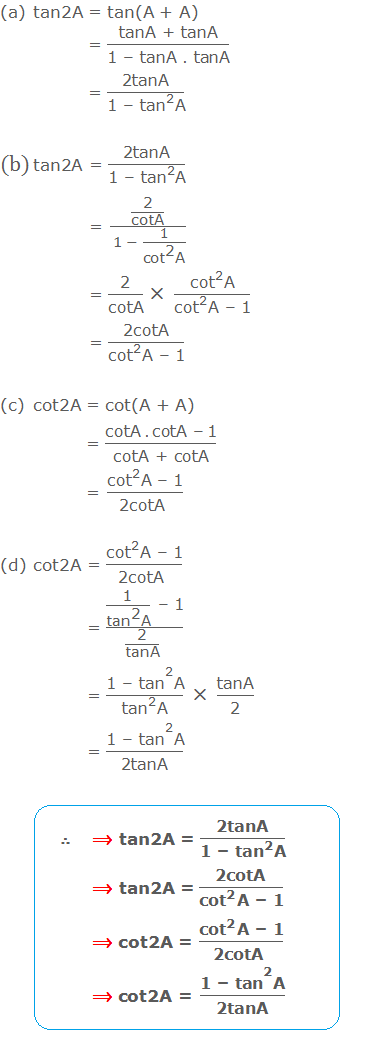#### Some Useful Results### Trigonometric Ratios of Angle 3A in terms of A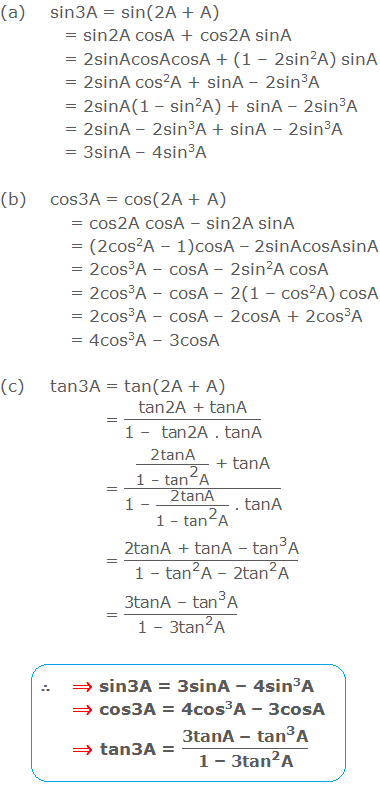### List of the trigonometric formula for multiple angles 2A and 3A: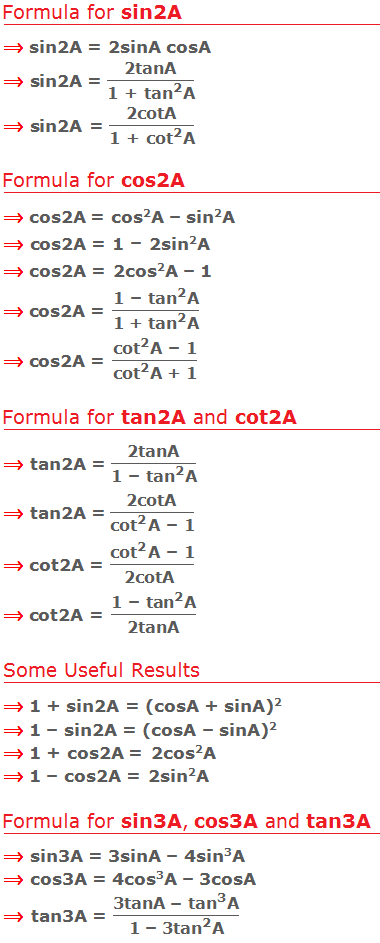### Geometrical Proof of 2A Formulae

Let O be the centre of the circle ABC and AB be a diameter (In the given figure below.). Let CAB = A, then COB = 2A [ Central angle is double of inscribed angle on same arc.]. ACB = 90° [Inscribed angle on semi-circle.]Let CM is perpendicular to AB. Then, ACM = 90° – A, and hence BCM = A.

Now from the right angle ΔOMC,### Worked Out Examples: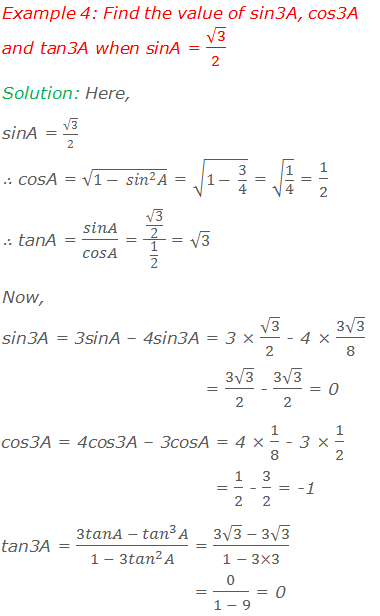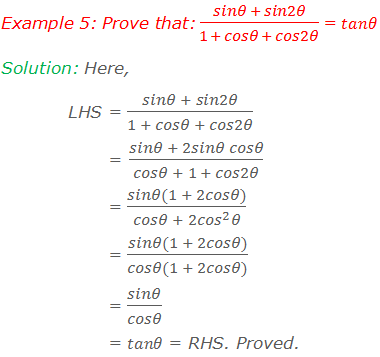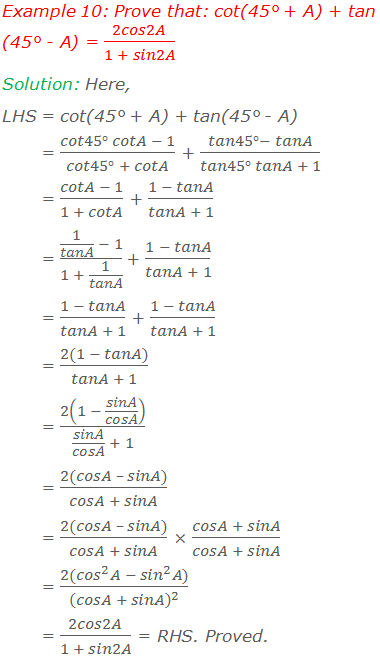#### Do you have any questions regarding the trigonometric ratios of multiple angles?

You can ask your questions or problems here, in the comment section below.